﻿ Statistical Simulations of Galactic Planetary Nebulae

Statistical Simulations of Galactic Planetary Nebulae

M. A. Sharaf, A. S. Saad, J. A. BasabrainOPEN ACCESSPEER-REVIEWED

Statistical Simulations of Galactic Planetary Nebulae

M. A. Sharaf1,, A. S. Saad2, 3, J. A. Basabrain4

1Department of Astronomy, Faculty of Science, King Abdulaziz University, Jeddah, KSA

2Department of Astronomy, National Research Institute of Astronomy and Geophysics, Cairo, Egypt

3Department of Mathematics, Preparatory Year, Qassim University, Buraidah, KSA

4Department of Statistics College of Science for Girls, King Abdulaziz University, KSA

Abstract

In the present paper, two catalogues J/A+A/327/736 and J/A+A/541/A98 of VizieR database were used for the statistical simulations of galactic planetary nebulae. Each catalogue was utilized for certain purposes, the first catalogue for, the correlation coefficients between the entireties of the data, the determination of the position of the maximum (minimum) of each entry in the data together with the values of the other entries at this position. In addition, we deuced the histograms and descriptive, location, dispersion and shape statistics for the entries of the data. Finally, the best fit and its error analysis was established between LH &LR where LH is the logarithm of HI Zanstrs luminosity and LR is the logarithm of nebular radius. The second catalogue was used to display two-dimensional distributions of the interacting planetary nebulae, moreover IAU and other references used the equatorial coordinates of these nebulae to determine the equatorial coordinates of the north galactic pole (NGP). The results of this application are in good agreement with those given.

At a glance: Figures

1
Prev Next

• Sharaf, M. A., A. S. Saad, and J. A. Basabrain. "Statistical Simulations of Galactic Planetary Nebulae." American Journal of Applied Mathematics and Statistics 3.2 (2015): 71-75.
• Sharaf, M. A. , Saad, A. S. , & Basabrain, J. A. (2015). Statistical Simulations of Galactic Planetary Nebulae. American Journal of Applied Mathematics and Statistics, 3(2), 71-75.
• Sharaf, M. A., A. S. Saad, and J. A. Basabrain. "Statistical Simulations of Galactic Planetary Nebulae." American Journal of Applied Mathematics and Statistics 3, no. 2 (2015): 71-75.

 Import into BibTeX Import into EndNote Import into RefMan Import into RefWorks

1. Introduction

A planetary nebula is created when a star blows off its outer layers after it has run out of fuel to burn. These outer layers of gas expand into space, forming a nebula, which is often the shape of a ring, bubble or other types ([4, 5, 11]). Only about 20% of planetary nebulae are spherically symmetric (for example, see Abell 39)  and likely produced by the old stars similar to the Sun . Planetary nebulae play a very important role in galactic evolution. The early universe consisted almost entirely of hydrogen and helium, but stars create heavier elements via nuclear fusion. In recent studies ([3, 9]), out of 200 billion stars, about 3500 planetary nebulae are now known to exist in our galaxy, more than double what it was a decade ago. They are found mostly near the plane of the Milky Way, with the greatest concentration near the galactic center .

The present paper, is devoted for the statistical simulations of galactic planetary nebulae, for such studies we used two catalogues and of VizieR database.

The first catalogue (its original reference is ) was utilized for establishing: (1) The correlation coefficients between the entireties of the data, (2) the position of the maximum (minimum) of each entry in the data together with the values of the other entries at this position, (3) the histograms of the entireties, (4) descriptive location, dispersion and shape statistics, (5) finally the best and its error analysis was developed between LH &LR where LH is the logarithm of HI Zanstrs luminosity and LR is logarithm of nebular radius.

The second catalogue (the original reference of the catalogue is ) was utilized for establishing the two dimensional distribution of the its nebulae and for determining the equatorial coordinates of the north galactic pole (NGP). The results of this application are in good agreements with those of IAU(1959),  and . A good review to the history of the Galactic coordinate system is given in .

2. Statistical Analysis of Planetary Nebulae Properties

For the present analysis, we used catalogue: of VizieR database (the original reference of the catalogue is ). What concerning us among the entries of the catalogue are the rows {6, 7, 8, 9, 10, 12, 16, 17}, the explanation of each row together with its used abbreviation are listed in Table 1.

2.1. Correlations between the entries

A correlation coefficient is a statistical measure of the degree to which two variables are related to each other. The linear correlation coefficient between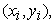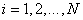is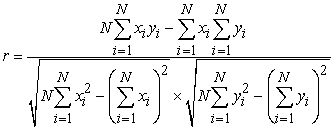(1)

This coefficient is a dimensionless quantity; that is, it does not depend on the units employed. Its value is always between, such that, the closest the value of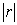to one is the more correlation between the two variables will be. The sign of r only fells us whether y is increasing or decreasing when x increases.

We find for the correlation coefficients between the entries of Table 1 (N=49), the values listed in Table 2.

Table 2. Correlation coefficients between the entries of Table 1

2.2. The Maxima and Minima

Table 3 (Table 4) gives the position of the maximum (minimum) of each entry in the data together with the values of the other entries at this position.

Table 4. The position of the minimum of each entry V and the values of the other entries at this position

2.3. The Histograms

The following figures show the histograms of the entries

2.4. Descriptive Statistics

The following are some descriptive statistics of the entries

2.4.1. Basic Descriptive Statistics
2.4.2. Location Statistics
2.4.3. Location Statistics

where,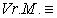variance of mean,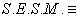standard error of sample mean,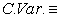coefficient of variation,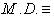mean deviation,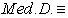median deviation and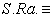sample range.

2.4.4. Shape Statistics 2.5. Best Fit between LH &LR and Its Error Analysis

1. We established for the best fit between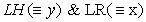the analytical representations: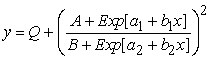(2)

where the coefficients and their probable errors are: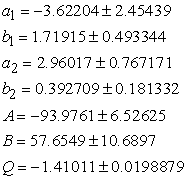2. The estimated variance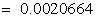.

3. Residuals at some points

4. The mean prediction confidence interval for some values of x are shown in the following table:

3. Galactic Pole Using Planetary Nebulae

For the present analysis, we used catalogue: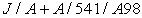of VizieR database (the original reference of the catalogue is ), hereafter will be referred to as Paper I). What concerning us among the entries of the catalogue, are the galactic coordinates and the equatorial coordinates ( right ascension & declination).

The sample used in Paper I is 117 planetary nebulae, the two dimensional distribution of the sample is shown in Figure 2.

Figure 2 confirms the result of Paper I in that, the majority of nebulae of the sample are located close to the galactic plane.

For the determination of the equatorial coordinates of the galactic pole,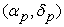we obviously shall except to get the best results choosing objects, which exhibit strong concentration towards the galactic plane. So the data of the sample of Paper I is suitable for such determination

3.1. Analytical Developments

It was shown that , the determination ofis a typical constrained minimization problem with:

1. the objective function is the sum of the N (say) squared distances of the selected objects from the plane of galactic plane.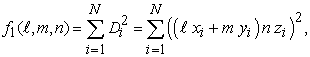(3)

where, on the assumption that, all objects having the equatorial coordinates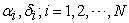are all at unit desistance from the Sun, so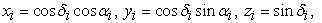(4)

and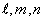are direction cosines of the perpendicular to the plane drawn from the origin.

2. The constrained of the problem is: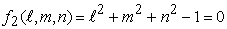(5)

Now, the solution of the constrained minimization problem is found by Lagrange's method so, the objective function becomes: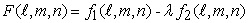(6)

where is Lagrange’s multiplier to be determined.

The necessary conditions for the minimum are: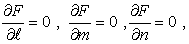(7)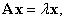(8)

where, the elements of the symmetric matrix A are given by: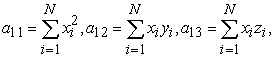(9)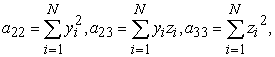(10)

It is well known that , the symmetric least square matrix A has all its eigen values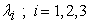real and distinct.

Now after solving the eigen value problem of Equation (8) for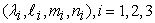select from this set, the values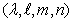that gives the global minimum of F, then the required values ofcould be determined from: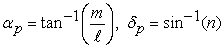(11)
3.2. Numerical Developments

Applying the above analytic developments for the data of Paper I, result as the average of five values corresponding to galactic latitudes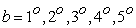of the members yields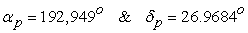.

In concluding the present paper, two catalogues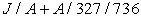andof VizieR database were used for the statistical simulations of galactic planetary nebulae. Each catalogue was utilized for certain purposes, the first catalogue for, the correlation coefficients between the entireties of the data, the determination of the position of the maximum (minimum) of each entry in the data together with the values of the other entries at this position. In addition, the histograms and descriptive, location, dispersion and shape statistics for the entries of the data are also developed. Finally, the best fit and its error analysis was established between LH &LR where LH is the logarithm of HI Zanstrs luminosity and LR is the logarithm of nebular radius.

The second catalogue was used to display two-dimensional distributions of the interacting planetary nebulae, moreover IAU and other references used the equatorial coordinates of these nebulae to determine the equatorial coordinates of the north galactic pole (NGP). The results of this application are in good agreement with those given.

References

  Ali A., Sabin L., Snaid S. and Basurah H. M., “Interacting planetary nebulae. I. Classification and orientation”, A & A 541, p. 1-10, 2012.In article  Jacoby, George. H., Ferland, Gary. J. and Korista, Kirk T., “The Planetary Nebula A39: An Observational Benchmark for Numerical Modeling of Photoionized Plasmas”, Ap. J. 560(1): 272-86, 2001.In article CrossRef  Kwitter, K. B. et al., “The Present and Future of Planetary Nebulae Research”, A White Paper by the IAU. Planetary Nebula Working Group, Revista Mexicana de Astronom´ıa y Astrof´ısica, 50, 203-223, 2014.In article  Kwok, Sun, and Su, Kate Y. L., “Discovery of Multiple Coaxial Rings in the Quadrupolar Planetary Nebula NGC 6881”, Ap. J. 635 (1): L49-52, 2005.In article CrossRef  Kwok, Sun, “The origin and evolution of planetary nebulae”, Cambridge University Press, 2000.In article CrossRef  Liu, J.-C., Zhu, Z. and Zhang, H., “Reconsidering the Galactic coordinate system”, A & A, 526 A16, p. 1-7, 2011.In article  Majaess, D. J., Turner, D. and Lane, D., “In Search of Possible Associations between Planetary Nebulae and Open Clusters”, PASP 119 (862): 1349-6, 2007.In article CrossRef  Osterbrock, Donald E. and Ferland, G. J., Ferland, G. J., ed., Astrophysics of gaseous nebulae and active galactic nuclei, University Science Books, 2005.In article  Parker, Quentin, A. et al., “The Macquarie/AAO/Strasbourg Hα Planetary Nebula Catalogue: MASH”, MNRAS, 373 (1): 79-94, 2006.In article CrossRef  Ralston, A. and Rabinowitz, P., “A First Course in Numerical Analysis”, McGraw-Hill Kogakusha, Ltd., Tokyo, 1978.In article  Rees, B. and Zijlstra, A. A., “Alignment of the Angular Momentum Vectors of Planetary Nebulae in the Galactic Bulge”, MNRAS, 435(2), 975-991, 2013.In article CrossRef  Sharaf, M. A., “Determination of a Celestial Plane Orientation: Application to Milky Way”, ROAJ, 18, no. 1, p. 61-67, 2008.In article  Schneider, P., “Extragalactic Astronomy and Cosmology: An Introduction”, Berlin, Springer, 2006.In article  Stasinska, G., Gorny, S. K, and Tylenda, R., “On the mass distribution of planetary nebulae central stars”, A & A, 327, p.736-742, 1997.In article  Martinez, Vicent J., and Saar, Enn, “Statistics of the Galaxy Distribution”, CRC Press, 2001.In article CrossRef